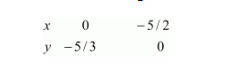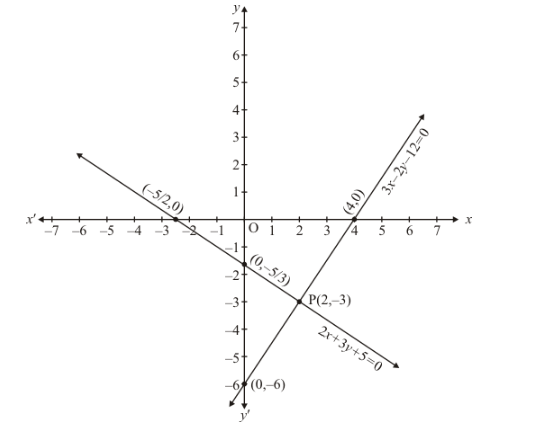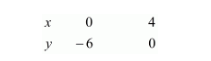# Solve the following systems of equations graphically:`
Question:

Solve the following systems of equations graphically:

$2 x+3 y+5=0$

$3 x-2 y-12=0$

Solution:

The given equations are

$2 x+3 y+5=0$$.(i) 3 x-2 y-12=0$$.(i i)$

Putting $x=0$ in equation $(i)$, we get:

$\Rightarrow 2 \times 0+3 y=-5$

$\Rightarrow y=-5 / 3$

$x=0, \quad y=-5 / 3$

Putting $y=0$ in equation $(i)$ we get:

$\Rightarrow 2 x+3 \times 0=-5$

$\Rightarrow x=-5 / 2$

$x=-5 / 2, \quad y=0$

Use the following table to draw the graph.Draw the graph by plotting the two points from table.Graph of the equation.... (ii):

$3 x-2 y=12 \quad \ldots \ldots \ldots .(i i)$

Putting $x=0$ in equation $(i i)$ we get:

$\Rightarrow 3 \times 0-2 y=12$

$\Rightarrow y=-6$

$x=0, \quad y=-6$

Putting $y=0$ in equation $(i i)$, we get:$\Rightarrow 3 x-2 \times 0=12$

$\Rightarrow x=4$

$x=4, \quad y=0$

Use the following table to draw the graph.Draw the graph by plotting the two points from table.

The two lines intersects at points $P(2,-3)$

Hence, $x=2$ and $y=-3$ is the solution.